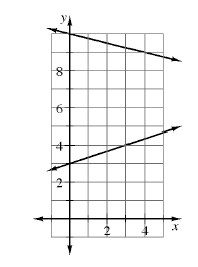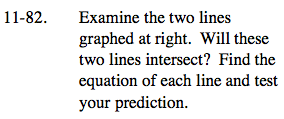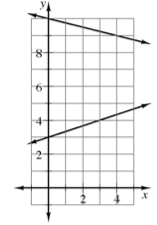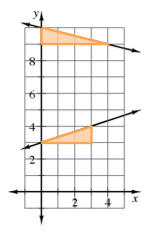Home > AC > Chapter 11 > Lesson 11.2.1 > Problem11-82

11-82.

Examine the two lines graphed below. Will these two lines intersect? Find the equation of each line and test your prediction. 11-82 HW eTool (Desmos). Homework Help ✎Find the slopes of each line by making slope triangles for each.
Look at the graph to determine the y-intercept for each line.

Use the Equal Values Method to solve the system.
Is there a solution?Use the eTool below to test your prediction.
Click the link at the right to view full version of the eTool: AC 11-82 HW eTool.